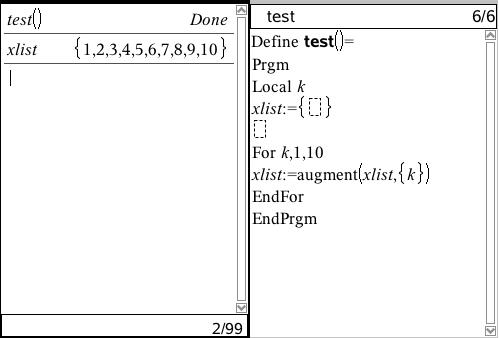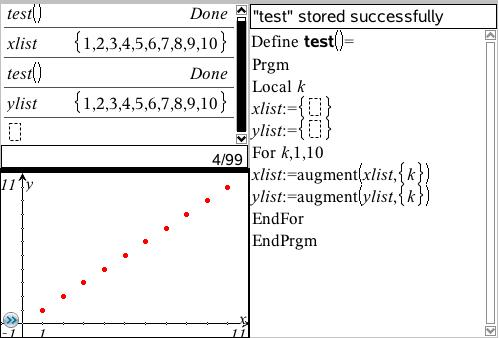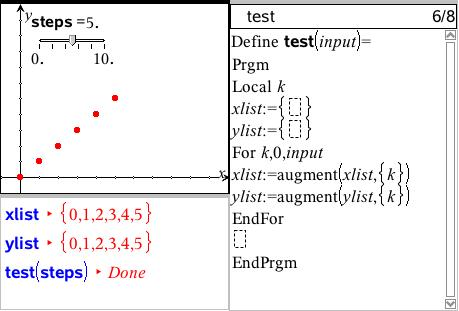©2010 Compass Learning TechnologiesHome TI-Nspire Authoring Dynamic Scatter Plots ← Programming Dynamic Scatter Plots

## Programming Dynamic Scatter Plots

The quickest way to define a list is to use the "sequence" command: so for the counting numbers from 1 to 10, seq(i,i,1,10) will produce the list {1,2,3,4,5,6,7,8,9,10}, while seq(i2,i,1,10} will result in the list {1,4,9,16,25,36,49,64,81,100}. This is fine for very simple number patterns, but quickly becomes confusing if more complicated lists are required. In this tutorial, we will be using a For...EndFor loop within a program to create our lists.

We can transform lists in certain simple ways. For example, if we have defined the list xlist:={1,2,3,4,5,6,7,8,9,10}, then

xlist+1 = {2,3,4,5,6,7,8,9,10,11}, while

2*xlist = {2,4,6,8,10,12,14,16,18,20}

However, if we tried xlist+{1,2,3} we would get an error, called a Dimension Mismatch, since to add or subtract lists to lists, these must have the same number of elements, or dimension. Thus, we could add xlist + {1,2,3,0,0,0,0,0,0,0} to get {2,4,6,4,5,6,7,8,9,10}.

Finally, to "glue" two lists together, we use the augment command: augment(list,{1,2,3}) = {1,2,3,4,5,6,7,8,9,10,1,2,3}

### And Now, some ProgramsDefine Prgm test()

Local k

xlist:={}

for k,1,10
xlist:=augment(xlist,{k})
endfor

EndPrgm

NOTE: We define the variable "k" as local so that it does not exist outside the actual program. It is just a counter and it is good practise to only create those variables that you actually need, so that there are not lots of variables floating around in the background. In this case, the only variable we want to exist outside the program is the list "xlist". Note also that the "augment" command takes two lists as inputs - the original one and the list of values you wish to append to it. We begin with xlist as an empty list; then the augment command adds new elements to it one at a time.

Having used this little program to create an xlist, where to from here?

Begin by doing the same to create a ylist.Define Prgm test()

Local k

xlist:={}
ylist:={}

for k,1,10
xlist:=augment(xlist,{k})
ylist:=augment(ylist,{k})
endfor

EndPrgm

We now have the ingredients for a scatter plot. Insert either a Data & Statistics page or a Graphs page and set up a scatter plot with our two lists, as shown. The D&S page is the quickest and easiest way to plot a scatter plot, but you have less control over the visual output and the Graphs page offers a wide range of useful additional tools and options, so it is generally the best option - especially if you are going to set the document up in advance.

So how to make our scatter plot dynamic? Insert a slider and call it something like "steps". Now change the program so that, instead of k running from 1 to 10, it runs from 1 to steps. Run the program n a MathBox in a Notes page (so that it is always running in the background) and set the input as your variable name, steps. Easy.Define Prgm test(input)

Local k

xlist:={}
ylist:={}

for k,1,input
xlist:=augment(xlist,{k})
ylist:=augment(ylist,{k})
endfor

EndPrgm

You now have a dynamic scatter plot - but while it runs in the Calculator, it does not seem very dynamic. So ditch the Calculator and run the program in a Notes page. It is that easy - now as you change the slider, your scatter plot changes along with it!

NOTE: What if you wanted to set up the program so that the user does not need to remember to enter an argument? makes life simpler, and programs (and functions) CAN pick up and use defined variables. But what if someone ran this program without first creating the variable "steps"? It would not work. In order to be safe, it is good to add a check to see if "steps" exists and, if not, to give it a value so that your program will work regardless. The command "getType" is perfect for this: If gettype(steps)≠"NUM":steps:=10. If steps exists, then it is a number, so getType checks this.The simplest and safest solution, however, is to require that any necessary variables are entered when you run the program, by making them arguments of the program. In the example shown, we define the program to require an argument, called "input". This can then be run using a specified value (for example, test(5)) or using a variable, as in our example, test(steps).

By including the required variable as the argument of the program, we ensure that the program cannot be run without a value being entered. Hence, there is no need to use the "gettype" test that we discussed above (this is still a very useful trick to have up your sleeve for other occasions though!).

Another useful change is to use the Attributes of the scatter plot to make it connected, as shown. This will generally be more effective. You might also wish to change the Attributes of the scatter plot so that the Points themselves are minimised, making it appear as a continuous line.

Now to make our scatter plot more interesting: what about constructing actual steps?

You need to think this through: we are going to construct a series of rectangles at each of the x-coordinates. They might begin with the first x point, go across 1 unit, then up the required height, then back one unit, then back to the starting point. Look closely at how this is achieved - the x and y coordinates are done separately, of course, so first you need to think in the x-direction, then in the vertical.Define Prgm test(input)

Try

Local k

xlist:={}
ylist:={}

for k,1,input
xlist:=augment(xlist,{k,k+1,k+1,k,k})
ylist:=augment(ylist,{0,0,k,k,0})
endfor

ex:="Steps up to "&string(input)

Else
ClrErr
EndTry

EndPrgm

How easy was that? Well, the more you do the easier it gets, so stick with it.

Did you notice that I added a string of dynamic text, which can be displayed in the Notes page? Press enter a few times to push the actual program down out of the way - the user does not need to see this. But it is often useful to display some accompanying text, so just define this as another variable and place it in a MathBox (set the MathBox Attributes to Hide Input).

Finishing the augmented list with a void element ("_") forces a break and creates discrete objects, which can be useful at times.

Finally, the Try..Else..Endtry block is very useful in programs. It serves as a safety switch - if anything goes wrong, then instead of an error message coming up and inviting the user to see where the error occurred in the program, it just ends the program.

### Some Challenges